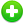#Australia Inflation Calculator

Rapidly calculate how the buying power of australian dollar has changed over time from year 1949 to present.

Year Dollar
In
In

If in 2010 You purchased an item for \$200 AUD then in 2021 that same item would cost \$247.06 AUD## How to Calculate the Australia Inflation Rate

Calculates the equivalent value of the Australia dollar in any year from 1949 to present. Calculations are based on the All Groups CPI, Weighted Average of Eight Capital Cities, Index Numbers (see the below table).This inflation calculator uses the following formula to calcualte the australia inflation rate.

Inflation Rate = ( CPI2 - CPI1 )CPI1 × 100

Where,

CPI - consumer price index.

CPI2 - The consumer price index in the second period.

CPI1 - The consumer price index in the previous period.

Australia Consumer Price Index (CPI) Table